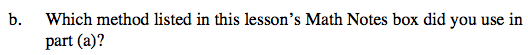### Home > CCA > Chapter 10 > Lesson 10.2.3 > Problem10-54

10-54.
1. For the equation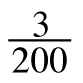+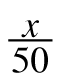=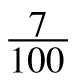: Homework Help ✎

1. Find a simpler equivalent equation (i.e., an equivalent equation with no fractions) and solve for x.

2. Which method listed in this lesson's Math Notes box did you use in part (a)?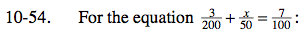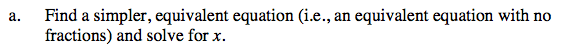Multiply the equation by a common multiple of the denominators to remove the fractions.

$200\left(\frac{3}{200}+\frac{x}{50}=\frac{7}{100}\right)$

3 + 4x = 14

Solve for x.